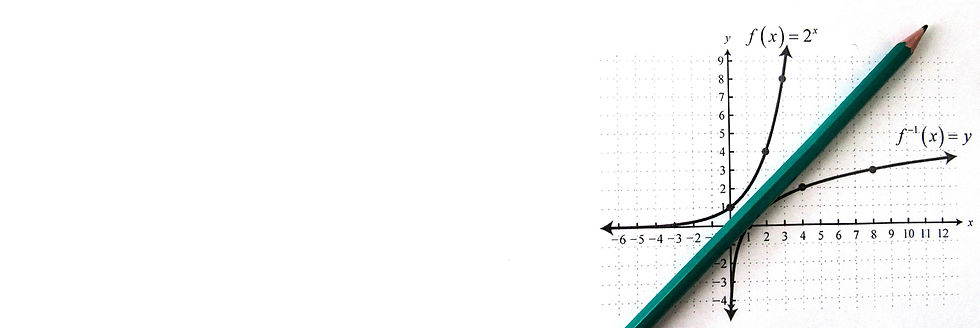###### 初级学院课程

We offer programmes for GCE 'A' level H1/H2 Mathematics syllabus.

###### Topic Outline H2 Mathematics
• Functions

• Graphs and transformations

•  Equations and inequalities

• Sequences and series

• Basic properties of vectors in two or three dimensions

• Scalar and vector products in vectors

• Three-dimensional vector geometry

• Complex numbers expressed in cartesian form

• Complex numbers expressed in polar form

• Differentiation

• Maclaurin series

• Integration techniques

• Definite integrals

• Differential equations

• Probability

• Discrete random variables

• Normal distribution

• Sampling

• Hypothesis testing

• Correlation and Linear regression

Each topic includes:

• Comprehensive revision notes

• Tutorials on questions on different level of difficulties.

• Challenging revision questions

Each term includes:

• Topical revision Papers

• Mock Exam/Test Papers

Students will:

• develop the ability to recognise similar type of questions

• learn the technique to comprehend exam questions and solve accordingly

• acquire the logical reasoning skill to solve questions

• recognise and apply Mathematical techniques accordingly in exam questions

# 9758 H2 Mathematics 2020 A-level Examination Syllabus

Paper 1

Duration: 3 hours

Number of questions: 10 to 12 questions of different lengths and marks based on the Pure Mathematics section of the syllabus

There will be at least two questions on application of Mathematics in real-world contexts, including those from sciences and engineering.

Each question will carry at least 12 marks and may require concepts and skills from more than one topic.

Candidates will be expected to answer ALL questions

Marks: 100

Weighting: 50%

Paper 2

Duration: 3hours

Consist of Section A and B

Section A (Pure Mathematics - 40 marks)

Number of questions: 4-5 questions of different marks and length.

Section B (Probability and Statistics - 60 marks)

Number of questions: 6-8 questions of different marks and length.

There will be at least two questions in Section B on application of Mathematics in real-world contexts, including those from sciences and engineering.

Each question will carry at least 12 marks and may require concepts and skills from more than one topic.

Candidates will be expected to answer ALL questions

Marks: 100

Weighting: 50%

##### 2019 GCE A level ResultEnquiry About Our Programmes or Registration